### Rate Equation from PFR data

The following conversion data were obtained in a tubular flow reactor for the gas-phase pyrolysis of acetone at 520oC and 1 atm.

CH3COCH3 -> CH3=C=O + CH4

```Flow rate, g/hr 	130	50	21	10.8
Conversion of acetone	0.05	0.13	0.24	0.35```

The reactor was 80 cm long with 3.3 cm inner diameter. What rate equation is suggested?

Calculations:

Volume of reactor = (π/4)D2 L = 684 cm3 = 0.684 lit

Molecular weight of acetone = 12 + 3 + 12 + 16 + 12 + 3 = 58

The above flow rate data in g/hr are converted into gmol/hr, by dividing them with Molecular weight.

Flow rate, g/hr 130 50 21 10.8

Molal flow rate, gmol/hr (FAo) 2.2414 0.8621 0.3621 0.1862

Conversion of acetone (XA) 0.05 0.13 0.24 0.35

For the (tubular flow) plug flow reactor the design equation is,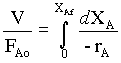1

CAo = pAo/RT = 1.01325 x 10-5/(8314 x (273 + 520))

= 0.01537 kmol/m3 = 0.01537 gmol/lit

For the first order reaction, (variable density systems)

-rA = kCA = kCAo(1 - XA)/(1 + εAXA) 2

substituting for -rA from equn.2 in equn.1,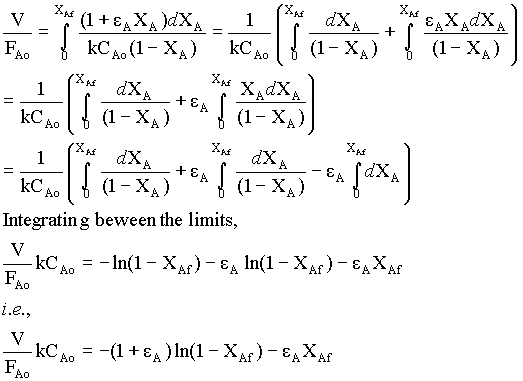for the given gas-phase reaction, εA = (VXA = 1 - VXA = 0) / VXA = 0 = (2 - 1)/1 = 1

Therefore,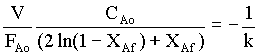For the constant V and CAo,

FAo ( 2 ln(1 - XAf) + XAf) = -VCAok = constant. 3

For second order reaction, (by Analytical integration)

k CAo2 V/FAo = 2εA(1 + εA) ln(1- XAf) + εA2XAf + (εA + 1)2 XAf/(1 + XAf)

For the given problem, εA = 1. Therefore,

(4 ln(1- XAf) + XAf + 4 XAf/(1 + XAf) ) FAo = k CAo2 V = constant. 4

We will check the above relations for various data available.

 Molal flow rate, gmol/hr (FAo) 2.2414 0.8621 0.3621 0.1862 Conversion of acetone (XAf) 0.05 0.13 0.24 0.35 For First order: FAo ( 2 ln(1 - XAf) + XAf) -0.11787 -0.12804 -0.11184 -0.09525 For Second order: (4 ln(1- XAf) + XAf + 4 XAf/(1 + XAf) ) FAo 0.124069 0.14712 0.146799 0.145369

From the tabulated data, it could be seen that the second order mechanism is well fitting the data, than first order.

Therefore, the reaction is following second order.

k CAo2 V = average of (4 ln(1- XAf) + XAf + 4 XAf/(1 + XAf) ) FAo for various FAo values

k CAo2 V = 0.146429 (obtained by omitting the first data)

Therefore, k = 0.146429 / (0.015372 x 0.684) = 906.2 lit/(gmol.hr)

And the equation is,

-rA = 906.2 CA2 gmol/(lit.hr)

Instead of analytical integration for 2nd order reaction, we can use numerical integration (Simpson's 1/3 rule)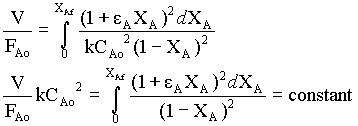Numerical integration is obtained for the function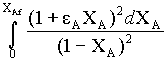by taking 10 intervals.

 Numerical Integration - by Simpson's rule 0.05 1 2 3 4 5 6 7 8 9 10 11 0 0.005 0.01 0.015 0.02 0.025 0.03 0.035 0.04 0.045 0.05 1 1.0202 1.0408 1.0618 1.0833 1.1052 1.1275 1.1503 1.1736 1.1974 1.2216 0.0554 0.13 0 1 2 3 4 5 6 7 8 9 10 0 0.013 0.026 0.039 0.052 0.065 0.078 0.091 0.104 0.117 0.13 1 1.0534 1.1096 1.1689 1.2314 1.2974 1.3670 1.4405 1.5182 1.6002 1.6870 0.1707 0.24 0 1 2 3 4 5 6 7 8 9 10 0 0.024 0.048 0.072 0.096 0.12 0.144 0.168 0.192 0.216 0.24 1 1.1008 1.2118 1.3344 1.4699 1.6198 1.7861 1.9708 2.1764 2.4057 2.6620 0.4054 0.35 0 1 2 3 4 5 6 7 8 9 10 0 0.035 0.07 0.105 0.14 0.175 0.21 0.245 0.28 0.315 0.35 1 1.1503 1.3237 1.5243 1.7572 2.0285 2.3459 2.7192 3.1605 3.6853 4.3136 0.7807

 XA Area FAo FAo XA Area 0.05 0.0554 2.2414 0.1241 0.13 0.1707 0.8621 0.1471 0.24 0.4054 0.3621 0.1468 0.35 0.7807 0.1862 0.1454